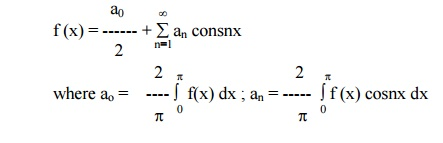# Even and Odd functions

A function f(x) is said to be even if f (-x) = f (x). For example x2(x square), cosx, x sinx, secx are even functions. A function f (x) is said to be odd if f (-x) = - f (x). For example, x3(x cube), sin x, x cos x,. are odd functions.

Even and Odd functions

A function f(x) is said to be even if f (-x) = f (x). For example x2, cosx, x sinx, secx are even functions. A function f (x) is said to be odd if f (-x) = - f (x). For example, x3, sin x, x cos x,. are odd functions.

(1) The Eulerâ€źs formula for even function is a(2) The   Eulerâ€źs   formula   for   odd   function   isExample 6

Find the Fourier Series for f (x) = x in ( -pp)

Here, f(x) = x        is an odd function.Example 7

Expand f (x) = |x| in (-pp) as FS and hence deduce thatExample 8Then find the FS for f(x) and hence show thatHere f (-x) in (-p,0) = f (x) in (0,p)

f  (-x) in (0,p) = f (x) in (-p,0)

f(x) is a even functionExample 9

Obtain the FS expansion of f(x) = x sinx in (-p< x<p) and hence deduce thatHere f (x) = xsinx  is an even function.Exercises:

Determine Fourier expressions of the following functions in the given interval:

i. f(x) = p/2 + x, -p< x < 0

p/2 - x, 0 < x < p

ii. f(x) = -x+1 for + -p< x < 0

x+1 for 0 =< x =< pStudy Material, Lecturing Notes, Assignment, Reference, Wiki description explanation, brief detail
Mathematics (maths) : Fourier Series : Even and Odd functions |